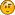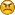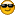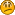# Math questions?

• 43 Replies
• 5671 Views
?

#### Harutsedo

• 1046##### Re: Math questions?
« Reply #30 on: June 14, 2011, 07:53:22 AM »
Prove that the derivative of e^x is e^x.

dx[ln(x)] = 1/x
dx[ln(e^x)] = dx[e^x]/e^x = dx[ x ] =1
For a fraction to be equal to one, both the numerator and denominator must be the same. Therefore, dx[e^x] = e^x

Man, e is awesome.
« Last Edit: June 14, 2011, 07:58:51 AM by Harutsedo »
Quote from: Tom Bishop
If you don't know, whenever you talk about it you're invoking the supernatural
Quote from: Tom Bishop
Unknown != Magic.#### hoppy

• Flat Earth Believer
• 11684##### Re: Math questions?
« Reply #31 on: June 14, 2011, 03:54:03 PM »
What is 33+ 17??

#### Thork##### Re: Math questions?
« Reply #32 on: June 14, 2011, 05:45:08 PM »
ooo. I have a question actually... so I have been trying to come up with a "nice" equation that describes the electric field inside the plane of a ring... my integrals keep getting reaaaaally ugly and I was wondering if you can come up with a nice integral for it...
http://hyperphysics.phy-astr.gsu.edu/hbase/magnetic/curloo.html#c1##### Re: Math questions?
« Reply #33 on: June 14, 2011, 06:31:27 PM »
ooo. I have a question actually... so I have been trying to come up with a "nice" equation that describes the electric field inside the plane of a ring... my integrals keep getting reaaaaally ugly and I was wondering if you can come up with a nice integral for it...
http://hyperphysics.phy-astr.gsu.edu/hbase/magnetic/curloo.html#c1
'thanks but that is the magnetic field at the center of a current loop. I was looking for the electric field as a function as how far we are from the center of the loop while still being in the plane.
You can't outrun death forever
But you can sure make the old bastard work for it.#### Tausami

• Flat Earth Editor
• 6767
• Venerated Official of the High Zetetic Council##### Re: Math questions?
« Reply #34 on: June 14, 2011, 06:51:43 PM »
Someone tell me how thick the ice wall would have to be to hold in the world's oceans. I did it myself, but there's a lot of room for error and I want to be double checked. I came up with about 5/8 of a mile.#### hoppy

• Flat Earth Believer
• 11684##### Re: Math questions?
« Reply #35 on: June 15, 2011, 06:46:56 AM »
What is 33+ 17?I'm still waiting. I thought this was just a troll thread.##### Re: Math questions?
« Reply #36 on: June 15, 2011, 07:06:41 AM »
Is the real part of any non-trivial zero in the Riemann zeta function 1/2?

Yes. All of them, in fact. I would write down the proof, but it is too large to fit in the margins.

That's the quote from Fermat's Small Theorem and not the Poincare and not suitable for the Riemann hypothesis.#### PizzaPlanet

• 12253
• Now available in stereo##### Re: Math questions?
« Reply #37 on: June 16, 2011, 08:41:54 AM »
It's funny because nobody writes equations that way. Math is a language, and writing 4+4/4 is just poor wording on the writer's part because it is ambiguous.
lrn2linear notation

dx[ln(x)] = 1/x
Hold your horses. ln(x)dx = 1/x is a direct result of e^xdx = e^x. You can't prove the rule with itself.
Also, (for some obscure reason) you've used the chain rule without proving its validity. That's no proof at all. It's just restating what you were supposed to prove.
« Last Edit: June 16, 2011, 08:50:47 AM by PizzaPlanet »
hacking your precious forum as we speak?

#### Harutsedo

• 1046##### Re: Math questions?
« Reply #38 on: June 16, 2011, 09:33:03 AM »
Hold your horses. ln(x)dx = 1/x is a direct result of e^xdx = e^x. You can't prove the rule with itself.
Also, (for some obscure reason) you've used the chain rule without proving its validity. That's no proof at all. It's just restating what you were supposed to prove.

I'll prove ln(x)dx = 1/x, too then. And what do you mean 'without proving its validity'? The chain rule has already been proven to work with any function, hasn't it?

e = lim (x->Math.huge) [(1 + 1/x)^x], by definition
dx[ln(x)] = lim (Δx->0) [(ln(x + Δx) - ln(x)) / Δx], by definition
ln(x) - ln(y) = ln(x/y)
dx[ln(x)] = lim (Δx->0) [(ln((x + Δx) / x)) / Δx]
dx[ln(x)] = lim (Δx->0) [(1 / Δx)(ln(1 + Δx/x)]

Let u = x / Δx
As Δx approaches infinite, u approaches 0.
1 / Δx = u / x
Δx / x = 1 / u

dx[ln(x)] = lim (u->infinite) [(u / x)(ln(1 + 1/u)], by substitution
dx[ln(x)] = lim (u->infinite) [(1 / x)ln(1 + 1/u)^u]
1/x is a constant, so
dx[ln(x)] = (1 / x)lim (u->infinite) [ln(1 + 1/u)^u]
From the limit of the composition of functions,...
dx[ln(x)] = (1 / x)ln(lim (u->infinite) [(1 + 1/u)^u])
lim (u->infinite) [(1 + 1/u)^u] = e, by definition
dx[ln(x)] = (1 / x)ln(e)
dx[ln(x)] = 1 / xQuote from: Tom Bishop
If you don't know, whenever you talk about it you're invoking the supernatural
Quote from: Tom Bishop
Unknown != Magic.

?

#### jackie cox

• 3##### Re: Math questions?
« Reply #39 on: June 16, 2011, 01:55:46 PM »
when, where, and how were diagonal matrices first stacked and integrated into the same math model creating a math model capable of combining an infinite number of variables into a single math platform, or the beginning of emperical matrix ?##### Re: Math questions?
« Reply #40 on: June 16, 2011, 02:36:29 PM »
when, where, and how were diagonal matrices first stacked and integrated into the same math model creating a math model capable of combining an infinite number of variables into a single math platform, or the beginning of emperical matrix ?

wut#### PizzaPlanet

• 12253
• Now available in stereo##### Re: Math questions?
« Reply #41 on: June 16, 2011, 09:23:35 PM »
e = lim (x->Math.huge) [(1 + 1/x)^x], by definition
This is not the definition of e. It's a derived equation. Derive it if you want to use it.
« Last Edit: June 16, 2011, 09:27:20 PM by PizzaPlanet »
hacking your precious forum as we speak#### Parsifal

• Official Member
• 36115
• Bendy Light specialist##### Re: Math questions?
« Reply #42 on: June 17, 2011, 05:34:11 AM »
when, where, and how were diagonal matrices first stacked and integrated into the same math model creating a math model capable of combining an infinite number of variables into a single math platform, or the beginning of emperical matrix ?

This is a history question. Go and create a history questions thread if you want to post things like this.
I'm going to side with the white supremacists.#### Johannes

• Flat Earth Editor
• 2755##### Re: Math questions?
« Reply #43 on: June 17, 2011, 12:24:50 PM »
diagonalize my avatar